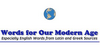### multiplicand[n] - the number that is multiplied by the multiplier
Found on http://www.webdictionary.co.uk/definition.php?query=multiplicand

### MultiplicandMul'ti·pli·cand` noun [ Latin multiplicandus to be multiplied: confer French multiplicande .] (Math.) The number which is to be multiplied by another number called the multiplier . See Note under Multiplication .
Found on http://www.encyclo.co.uk/webster/M/114

### multiplicandnoun the number that is multiplied by the multiplier
Found on https://www.encyclo.co.uk/local/20974

### Multiplicand• (n.) The number which is to be multiplied by another number called the multiplier. See Note under Multiplication.
Found on http://thinkexist.com/dictionary/meaning/multiplicand/

### multiplicand(from the article `arithmetic`) ...is independent of the way in which the summands are grouped; it can be written 3 5. Thus, a second binary operation called multiplication is ...
Found on http://www.britannica.com/eb/a-z/m/137

### multiplicandmultiplicand A number that is multiplied by another number (the multiplier).
Found on http://www.wordinfo.info/words/index/info/view_unit/1701/5
No exact match found